All You Need to Know About

# Array Formula in Excel

The Excel Array Formula lets you performs math, comparative, join operations on an array or range of data. It can seem overwhelming at first but it does not have to be! Read on to find out how you can use the Array Function in Excel to the fullest!

Here are the top things on what you can do with the Array Formula in Excel:

## Excel Array Formulas Explained

An Array Formula performs an Excel operation (math, comparative, join or function argument) on an array or range of data.  This could be a range of cells, a worksheet reference or a defined name.
An Array contains more than one cell, so you cannot perform an Array Formula on a single cell.
To turn your formula into an Array Formula, you will need to press CTRL+SHIFT+ENTER which will put the squiggly brackets {} outside the formula:

{=MAX(D13:D16C13:C16)}

Let’s break down the different Excel operations that you can use to create an Array Formula:
MATH: +-*/()
COMPARATIVE: =
JOIN: &
FUNCTIONS: MAX, MIN, IF, INDEX, VLOOKUP etc

Let’s do an example of an Array Formula that calculates the maximum stock value change over a four day period:

STEP 1: Enter the MAX formula =MAX(
STEP 2: Subtract one array/range of data from another array/range of data =MAX(D13:D16C13:C16)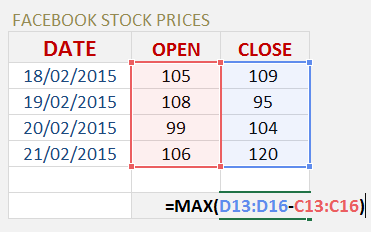STEP 3: Instead of pressing ENTER to evaluate the formula, you need to press CTRL+SHIFT+ENTER to turn the formula into an Array Formula which will look like this: {=MAX(D13:D16C13:C16)}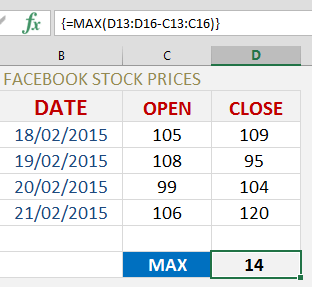STEP 4: By pressing F9 on the selected formula array will give you the resulting array of numbers (press CTRL+Z to get out of this mode when you are done checking the formula results):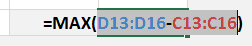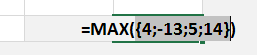If we had to get the above result using a non-Array Formula we would have to create a helper column which subtracts the Open & Close cells and then enter the MAX formula to reference these results.  This is double the work!

## Calculate Total Sales With An Array Formula

An Array Formula performs an Excel operation (math, comparative, join or function argument) on an array or range of data.  This could be a range of cells, a worksheet reference or a defined name.
One of the most popular uses of an array formula is to calculate Total Sales.
If we had to calculate Total Sales the normal way, we would have to create a “helper column” for the Totals column and then enter a formula to Sum all the Totals.
Using an Array Formula we skip the “helper column” and just enter one formula only.  Quick and simple!
Let’s do an example of an Array Formula that calculates the Total Sales for various products:

STEP 1: Enter the SUM formula =SUM(
STEP 2: Select the array/range of data for the UNITS SOLD, enter the multiplier sign * and select the array/range of the data for the UNIT PRICE:
=SUM(C13:C16*D13:D16)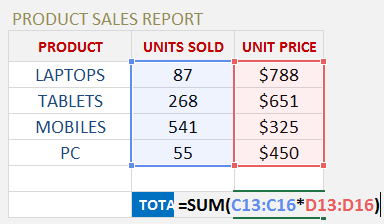STEP 3: Instead of pressing ENTER to evaluate the formula, you need to press CTRL+SHIFT+ENTER to turn the formula into an Array Formula which will look like this:
{=SUM(C13:C16*D13:D16)}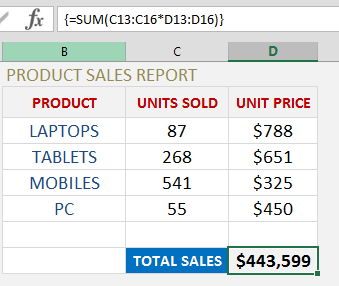STEP 4: By pressing F9 on the selected formula array will give you the resulting array of numbers (press CTRL+Z to get out of this mode when you are done checking the formula results):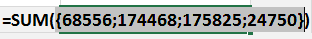If we had to get the above result using a non-Array Formula we would have to create a helper column which multiplies UNITS SOLD by UNIT PRICE and then enter the SUM formula to get the same result.  This is double the work!

## Total Bonus Due With An Array Lookup Formula

The LOOKUP function has three arguments, it is a like a simplified VLOOKUP function:
What does it do?
It looks up a value (lookup_value) in one range (lookup_vector) and returns a value from the same position in a second range (result_vector)
Formula breakdown:
=LOOKUP(lookup_value, lookup_vector, result_vector)
What it means:
=LOOKUP(The value that you want to lookup, Range that contains the values to be lookup up, Range that contains the values to be returned)
** Note that the LOOKUP function uses the Approximate Match argument automatically (TRUE argument in a VLOOKUP), so therefore the result_vector has to be sorted in ascending order **
Read this blog that explains the VLOOKUP using the Approximate or TRUE argument

Say that you want to calculate the Total Bonuses due to the Sales Reps, you can do that using the SUM(LOOKUP()) formula and then pressing CTRL+SHIFT+ENTER to turn it into an Array formula.
You will need to have your data set up into three separate columns, one for the Lookup Values (Sales made by the Sales Rep), another for the Lookup Range (Sales bonus threshold) and the last one for the Results Range (Bonus payable in ascending order)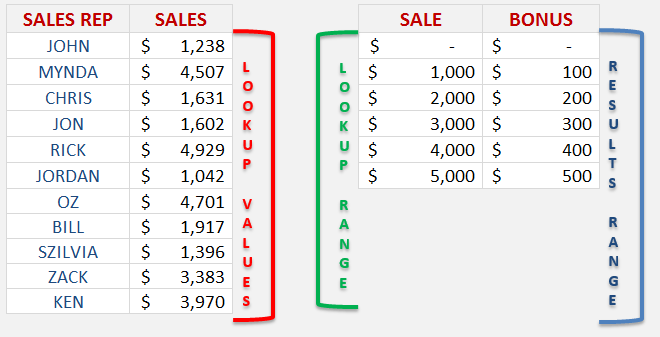STEP 1: Enter the SUM function =SUM(
STEP 2: Nest the LOOKUP function =SUM(LOOKUP(
STEP 3: Select the Lookup Values in the Sales Rep Sales column:
=SUM(LOOKUP(C13:C23,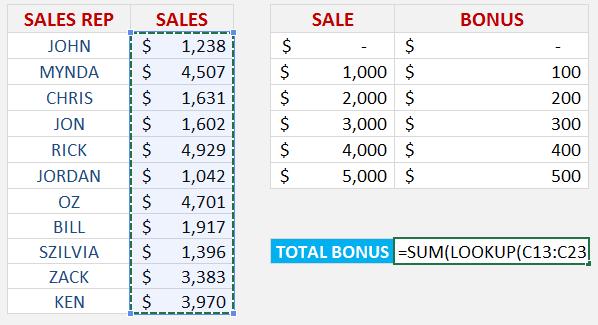STEP 4: Select the Lookup Range in the Sale bonus threshold column:
=SUM(LOOKUP(C13:C23,E13:E18,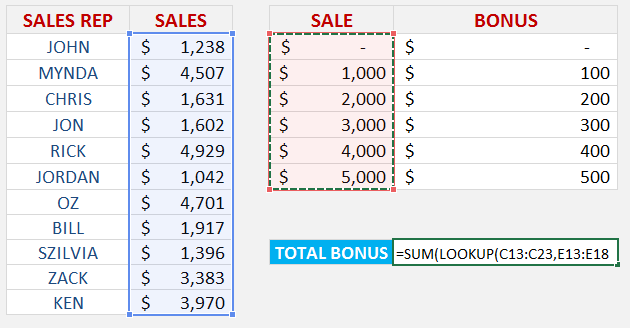STEP 5: Select the Results Range in the Bonus payable column:
=SUM(LOOKUP(C13:C23,E13:E18,F13:F18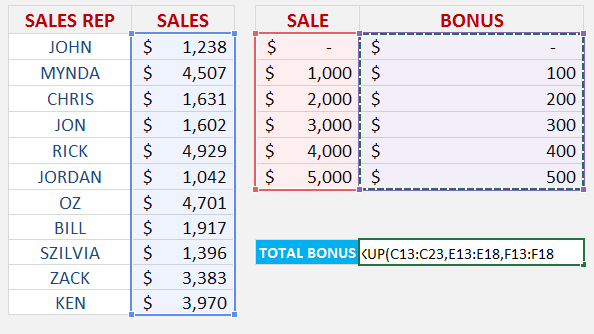STEP 6: Instead of pressing ENTER to evaluate the formula, you need to press CTRL+SHIFT+ENTER to turn the formula into an Array Formula which will look like this:
{=SUM(LOOKUP(C13:C23,E13:E18,F13:F18))}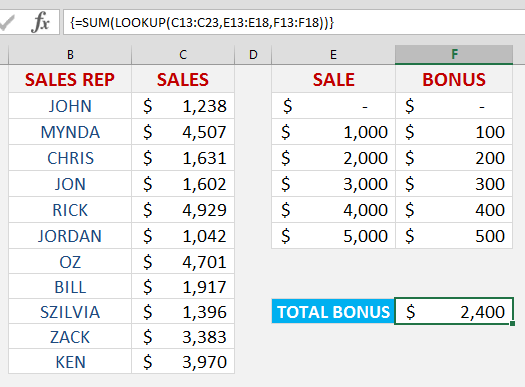STEP 7: By pressing F9 on the selected formula array will give you the resulting array of numbers that get summed up (press CTRL+Z to get out of this mode when you are done checking the formula results):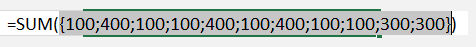Latest Tutorials

March 3, 2021

June 26, 2020

## Total Bonus Due With An Array Lookup Formula

February 24, 2016

## Excel Array Formulas Explained

December 16, 2015